The LAMBDA function in EXCEL 365 will revolutionize the way you can build custom functions in Excel.

You can create a custom function in Excel without the use of VBA user-defined functions.

## Want to know how to use the LAMBDA function?

*** Watch our video and step by step guide below with free downloadable Excel workbook to practice ***

Watch it on YouTube and give it a thumbs-up!

### LAMBDA function in Excel

What is this newly introduced function – Excel LAMBDA???

The LAMBDA function in Excel can be used to create a custom function without any coding and then reuse it anywhere in your spreadsheet. This Excel function is exclusively available in Excel 365 only.

At the time of publishing this article, it is being rolled out slowly around the world for Office Insiders who are signed up for Beta Channel (You can check what Excel version you have here).

You can use Excel’s own formula language to create custom functions. So, no need to learn complex coding language like VBA.

You can call another function in this function as well. Hence, there is no limit to the power of this super useful function.

Let’s take a quick look at the syntax of this function!

### Syntax of LAMBDA function

The LAMBDA function syntax has the following arguments:

### =LAMBDA(parameter1, parameter2, …, calculation)

• parameter(s) – The value you want to use in the calculation. It can be a cell reference, range, or number. There can be numerous parameters in this function.
This argument is optional.
• calculations – The formula you want Excel to execute. It should be the last argument of the function.
This argument is required.

Let’s look at an example and understand how this function works.

### Examples of LAMBDA function

Example 1:

Suppose you have the formula

### =LAMBDA(start,end,end/start-1)

Here,

• start and end are the parameters
• end/start-1 is the calculation

### Calling the LAMBDA function

We need one more step to conclude our LAMBDA function.  If you skip this step, you will get #CALC! error.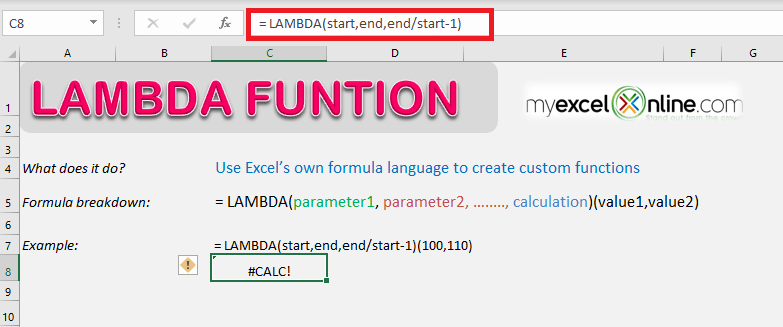In our example above, we need to enter the values for “start” and “end” within the parenthesis to call this function and get a result:

### =LAMBDA(start,end, end/start-1)(100,110)

Here,

• 100 is the value that will be used for start
• 110 is the value that will be used for end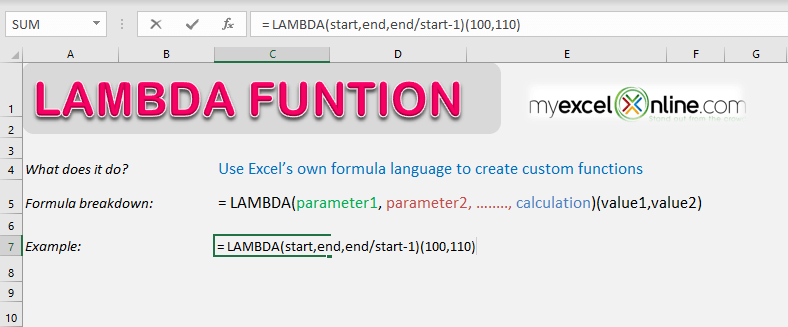Excel will now replace the parameters with the valued and complete the calculation as:

=110/100-1

=0.10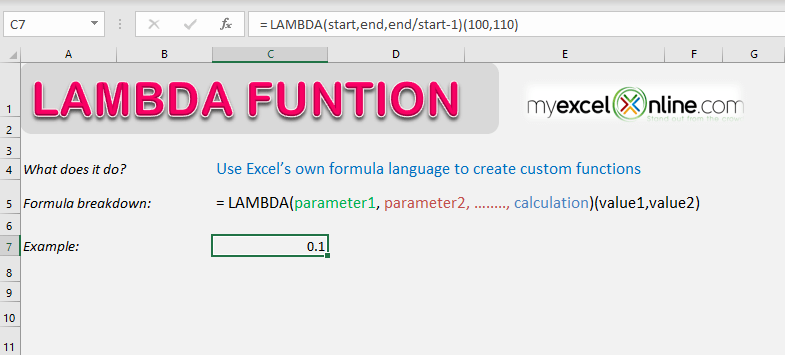Example 2:

In the example below, we have a column with the pound values and a column with the conversion rate.

In the third column, we will be using the LAMBA function to create a custom function to convert the amount from pound to dollar using the conversion rate.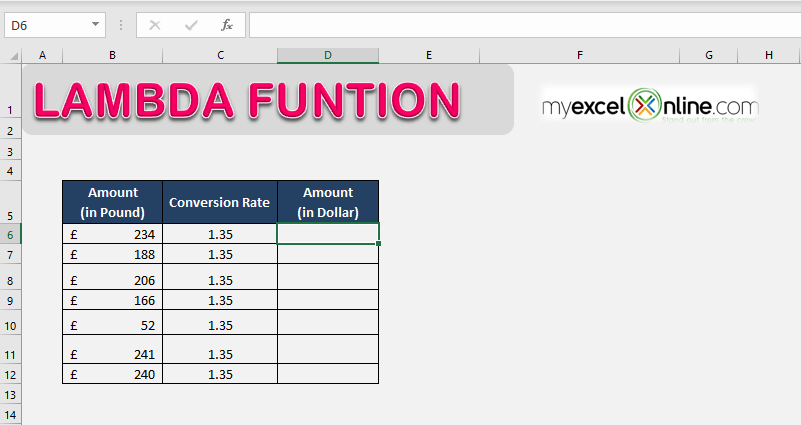Follow the step-by-step tutorial below to know how to create custom functions in Excel using the LAMBA function:

STEP 1: Start with an equal to sign and type the name of the formula.

### =LAMBDA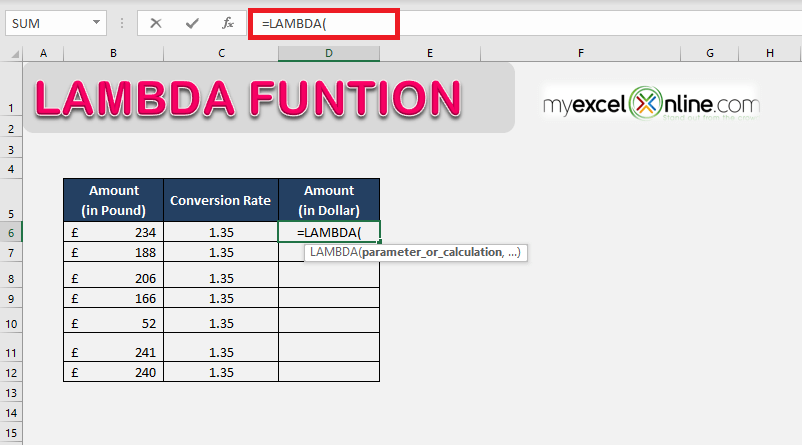STEP 2: Open parenthesis and type the first argument of the function i.e. The parameters (amount in pound and conversion rate)

### =LAMBDA (pound, conversion,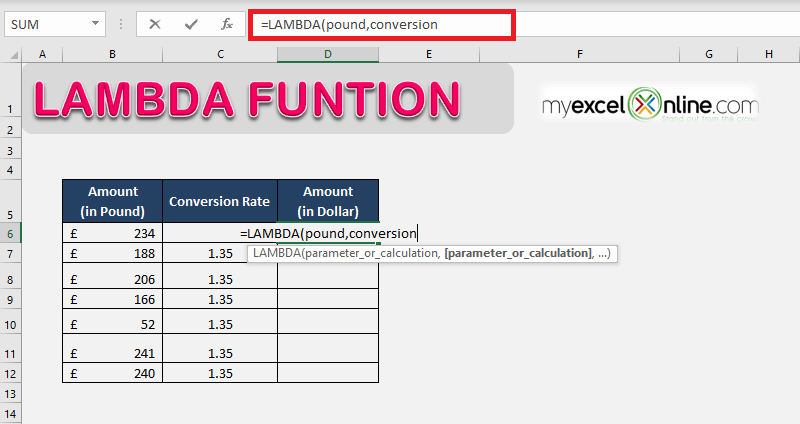STEP 3: Now, enter the custom calculation i.e. pound*conversion, and close the parenthesis.

### =LAMBDA (pound, conversion, pound*conversion)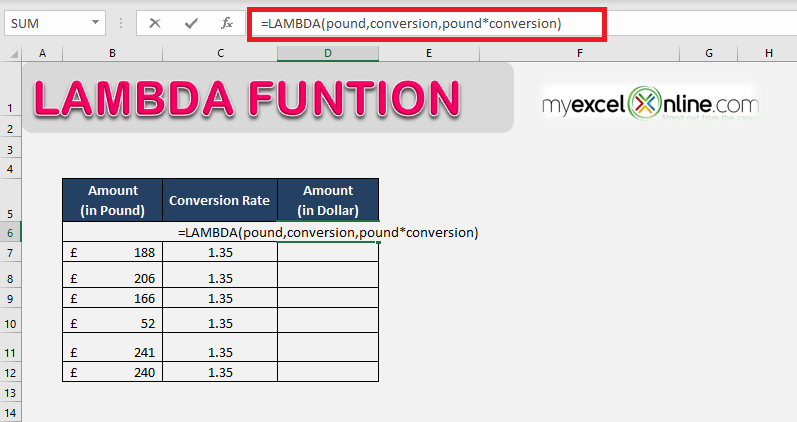STEP 4: Next, you need to provide the inputs for this function within another parenthesis so we can get the results (If you skip this step, you will get #CALC! error).

### =LAMBDA (pound, conversion, pound*conversion)(B6,C6)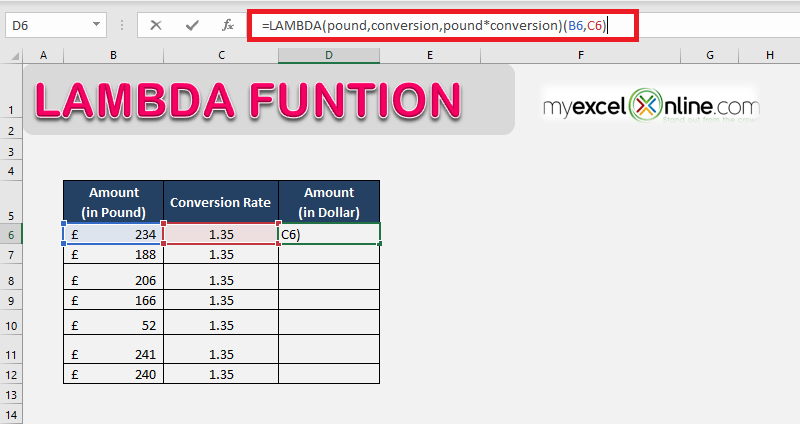STEP 5: The converted value in dollar will be displayed in the cells when you drag the formula all the way down.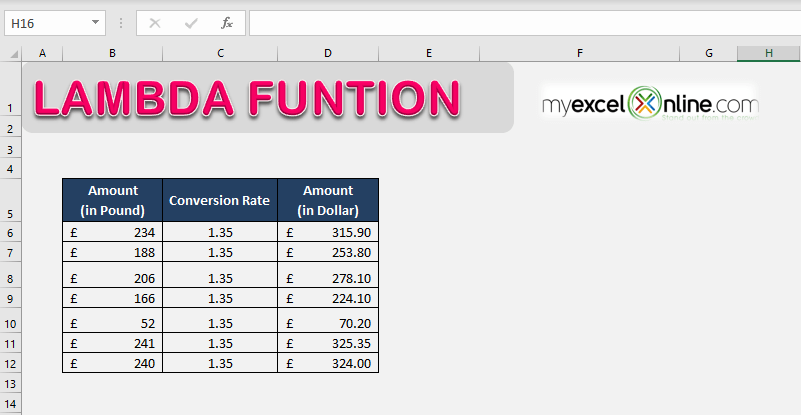### Naming a LAMBDA function in Excel

THIS IS WHERE THE POWER OF THE LAMBDA FUNCTION EXISTS!

Now that you have learned how to create the new LAMBDA function in Excel, let’s move forward and take a look a how you can store this function in Excel so that you can re-use it within your Excel workbook.

Follow the steps below to use Name Manager to store the LAMBDA function (created in Example 2) for re-use.

STEP 1: Go to Formulas > Name Manager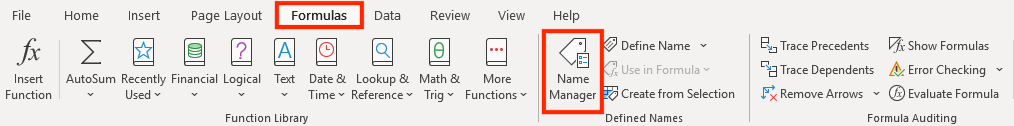STEP 2: In the Name Manager dialog box, click on the New button.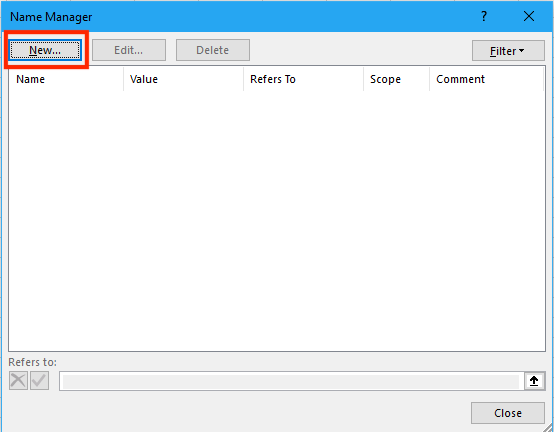STEP 3: In the New Name dialog box, enter the following details

• Name of the function i.e. CurrencyConversion (Make sure there are no spaces)
• Brief description related to this function i.e. Use this function to convert pound to dollar.
• The parameters of the function i.e. =LAMBDA(pound,conversion,pound*conversion).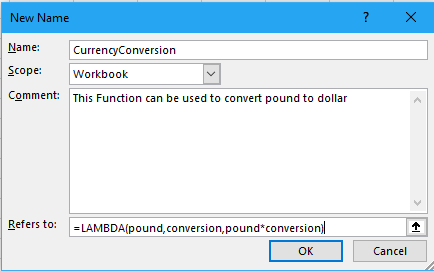STEP 4: Click OK.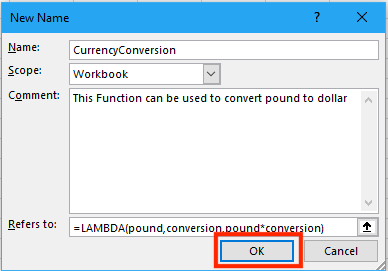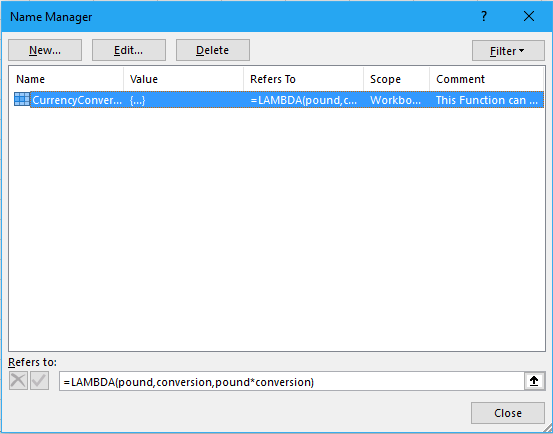You can use this custom function by calling the function name – CurrencyConversion.

### =CurrencyConversion(316,1.4)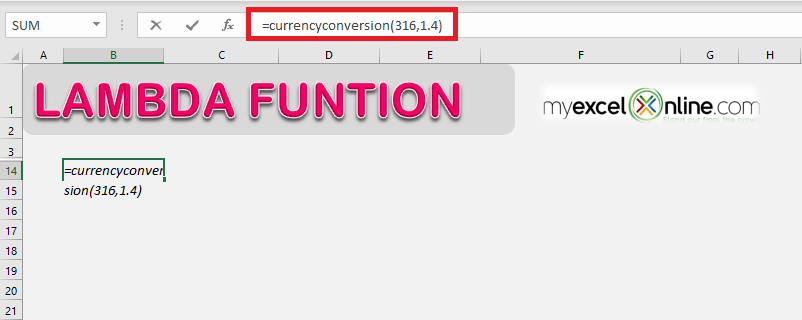Excel will return the value 442.2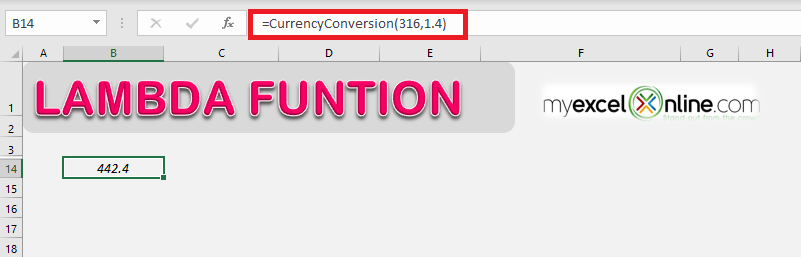This AWESOME function is available for Microsoft 365 users only who are on the Office Insider Beta program and will be rolled out gradually to the rest of the programs in the coming months. Click here to purchase Microsoft 365.

You can also go through the Microsoft tutorial on the LAMBDA function in Excel by clicking here!

Make sure to download our FREE PDF on the 333 Excel keyboard Shortcuts here:You can learn more about how to use Excel by viewing our FREE Excel webinar training on Formulas, Pivot Tables, Power Query, and Macros & VBA!